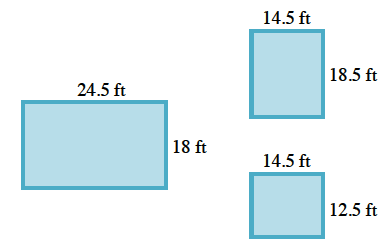### Home > CC1 > Chapter 5 > Lesson 5.2.1 > Problem5-49

5-49.

Melissa wants to re-sod her yard (replace the grass). Her backyard has a rectangular lawn area that measures $24\frac{1}{2}$ feet by $18$ feet. Her front yard has two rectangular areas, one of which measures $18\frac{1}{2}$ feet by $14\frac{1}{2}$ feet. The other measures $12\frac{1}{2}$ feet by $14\frac{1}{2}$ feet. How many square feet of sod does Melissa need? Show all of your work clearly. Homework Help ✎

Drawing a diagram like the one below should be helpful in this problem.